AspirinCE
AspirinCE is a fun reaction game with three difficulty levels and 3 different highscores.Hello again! So while I take a break from DoodleJump, I kinda got distracted and am now attempting to port Aspirin. So far I've got one of those stabby lines moving, a player, and one of the point balls.Bumpity bump!

I've gotten collision for both circles and lines working, and I just need to implement more lines, now. Anyone have an idea for that? I'm thinking maybe a matrix? I'm not sure. Any suggestions would be great!So yeah, got more lines and things, but at a certain point things slow down: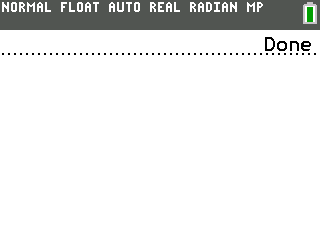So to counteract that, Mateo suggested drawing over the lines with white versions of themselves instead of redrawing the whole screen. Here's what that looks like. Any idea how to make it erase all the black?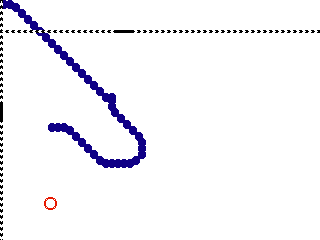Code:
``` //-------------------------------------- // Program Name: // Author: // License: // Description: //-------------------------------------- /* Keep these headers */ #include <stdbool.h> #include <stddef.h> #include <stdint.h> #include <tice.h> /* Standard headers - it's recommended to leave them included */ #include <math.h> #include <stdio.h> #include <stdlib.h> #include <string.h> #include <graphx.h> #include <keypadc.h> #include <fileioc.h> #include <debug.h> /* Other available headers */ // stdarg.h, setjmp.h, assert.h, ctype.h, float.h, iso646.h, limits.h, errno.h, debug.h /* Put your function prototypes here */ void renderGame(); void drawLines(); void checkForStab(); void updateLines(); void addSixLines(); /* Put all your globals here. */ int playerX = 4, playerY = 4; uint8_t numLines = 66, enabledLines; bool quit; typedef struct my_line {    int x;    int y;    bool direction;    bool displaying;    bool goingRight; } my_line_t; my_line_t lines; void main(void) {    int key = 0, pointX = 0, pointY = 0, i = 0;    bool pointGrabbed = true;    srand(rtc_Time());    gfx_Begin(gfx_8bpp);    gfx_FillScreen(gfx_white);    gfx_SetDrawBuffer();    for (i = 0; i<numLines; i++) {       lines[i].y = 30;    }    for (i = 0; i<numLines; i++) {       lines[i].displaying = true;       lines[i].y = 30;       lines[i].goingRight = true;    }    while (kb_ScanGroup(kb_group_6) != kb_Clear) {       checkForStab();       renderGame();       if (pointGrabbed) {          pointX = randInt(1,328);          pointY = randInt(1,208);          addSixLines();          pointGrabbed = false;       }       if (pointGrabbed == false) {          gfx_SetColor(gfx_red);          gfx_Circle(pointX, pointY, 6);          gfx_Circle(pointX, pointY, 5);       }       if (sqrt((playerX-pointX)*(playerX-pointX) + (playerY-pointY)*(playerY-pointY)) <= 10 && sqrt((playerX-pointX)*(playerX-pointX) + (playerY-pointY)*(playerY-pointY)) >= 0) {          pointGrabbed = true;       }       gfx_SwapDraw();       if (key & kb_Up && playerY >= 10)          playerY -= 3;       if (key & kb_Down && playerY < 235)          playerY += 3;       if (key & kb_Left && playerX > 4)          playerX -= 3;       if (key & kb_Right && playerX < 315)          playerX += 3;       key = kb_ScanGroup(kb_group_7);       if (quit == true)          break;    }    gfx_End();    pgrm_CleanUp(); } void renderGame(void) {    //gfx_FillScreen(gfx_white);    drawLines(gfx_white);    updateLines();    drawLines(gfx_black);    gfx_SetColor(gfx_blue);    gfx_FillCircle(playerX, playerY, 4); } void drawLines(uint8_t color) {     uint8_t i = 0;     gfx_SetColor(color);     if (lines.displaying) {         for (i = 0; i < enabledLines; i++) {             my_line_t *this_line = &lines[i];             int x = this_line->x;             int y = this_line->y;               if (this_line->displaying) {                 if (!this_line->direction) {                     gfx_FillRectangle(x, y, 20, 3);                     gfx_SetPixel(x-1, y+1);                     gfx_SetPixel(x+20, y+1);                 } else {                     gfx_FillRectangle(x, y, 3, 20);                     gfx_SetPixel(x+1, y-1);                     gfx_SetPixel(x+1, y+20);                 }             }         }     } } void checkForStab(void) {    int i = 0;    /*for (i = 0; i<8; i++) {       if (gfx_GetPixel(playerX-6+i, playerY-5) == gfx_black)          quit = true;    }    for (i = 0; i<8; i++) {       if (gfx_GetPixel(playerX+5, playerY-6+i) == gfx_black)          quit = true;    }    for (i = 0; i<8; i++) {       if (gfx_GetPixel(playerX+6-i, playerY+5) == gfx_black)          quit = true;    }    for (i = 0; i<8; i++) {       if (gfx_GetPixel(playerX-5, playerY+6-i) == gfx_black)          quit = true;    }*/ } void updateLines(void) {     uint8_t i;     if (lines.displaying == true) {         for(i = 0; i < enabledLines; i++) {             my_line_t *this_line = &lines[i];             int x = this_line->x;             int y = this_line->y;               if (!this_line->direction) {                 if (x <= 0) {                     this_line->goingRight = true;                 }                 if (x >= 310) {                     this_line->goingRight = false;                 }                 if (this_line->goingRight) {                     this_line->x +=3;                 } else {                     this_line->x -=3;                 }             } else {                 if (y <= 0) {                     this_line->goingRight = true;                 }                 if (y >= 235) {                     this_line->goingRight = false;                 }                 if (this_line->goingRight) {                     this_line->y +=3;                 } else {                     this_line->y -=3;                 }             }        }    } } void addSixLines() {    int i;    enabledLines += 6;    for (i=0; i<enabledLines; i++) {       lines[i].direction = randInt(0,1);       lines[i].displaying = true;    }    dbg_sprintf(dbgout, "Lines On Screen: %d\n", enabledLines); } /* Put other functions here */ ```

This looks awesome, and i really wish i could help you, but i know nothing other than BASIC!!!Without closely-inspecting your code, are you erasing your lines before you move them?
• It looks like you might be moving them, then erasing them, leaving a trail behind.
• If you're moving them, then erasing them, and offsetting the erasure by how much the lines moved, are you sure you're doing so correctly?
• I definitely don't recommend that latter method (and if you do erase after computing the new positions, I might suggest saving the original positions instead of trying to guess them).
It's because of the two buffers. When you are drawing you should *ahem* draw everything twice if you want to use this buffering method. Once you draw the first render, swap it to the visible screen. Then (on the back buffer) draw everything again, and swap again. Then erase the lines in the buffer, draw the new ones in the buffer, and swap. Then erase the lines in the buffer, draw the new ones in the buffer, and swap. And that should solve the buffering issues.

An alternate (easier, but might be a little slower) would be to just draw everything in the back buffer, and then call gfx_BlitBuffer(), rather than gfx_SwapDraw().( Credits to Rico for pointing this out earlier)
Also your enabledLines variable isn't initialized ever. That could cause errors in the future.
Welp, thanks for the pointers everyone! I found a problem with coordinates not being randomized correctly. and lines being drawn over themselves, so I fixed that, and now it is much harder before the slowdown occurs. I am also going to make the area where the lines and the player move into a smaller square so I don't have to draw as much, speeding up the program a bitHere's a screenshot for now. (Notice 6 lines instead of 2 in the beginning)So yeah, gonna add a menu and more levels after that. 6 per point is going to be the hardest, I think.
bump.

So, beside the fact that my code is pretty unoptimized, could anyone try figuring out why when I get a point the coordinates for already displaying lines gets reset, and displays in another place? I'm thinking a fresh set of eyes could help.Watch Closely: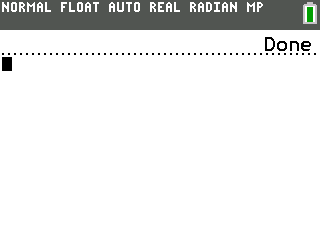Code:
``` //-------------------------------------- // Program Name: // Author: // License: // Description: //-------------------------------------- /* Keep these headers */ #include <stdbool.h> #include <stddef.h> #include <stdint.h> #include <tice.h> /* Standard headers - it's recommended to leave them included */ #include <math.h> #include <stdio.h> #include <stdlib.h> #include <string.h> #include <graphx.h> #include <keypadc.h> #include <fileioc.h> #include <debug.h> /* Other available headers */ // stdarg.h, setjmp.h, assert.h, ctype.h, float.h, iso646.h, limits.h, errno.h, debug.h /* Put your function prototypes here */ void drawLines(); void checkForStab(); void updateLines(); void addSixLines(); /* Put all your globals here. */ int playerX = 4, playerY = 4; uint8_t numLines = 66, enabledLines; bool quit; typedef struct my_line {    int x;    int y;    bool direction;    bool displaying;    bool goingRight; } my_line_t; my_line_t lines; void main(void) {    int key = 0, pointX = 0, pointY = 0, i = 0, keypress = 0;    bool pointGrabbed = false;    srand(rtc_Time());    gfx_Begin(gfx_8bpp);    gfx_FillScreen(gfx_white);    gfx_SetDrawBuffer();    for (i = 0; i<numLines; i++) {       my_line_t *this_line = &lines[i];       this_line->y = 30;       this_line->goingRight = true;    }    while (kb_ScanGroup(kb_group_6) != kb_Clear) {       if (keypress % 1 == 0) {             //Erase Screen          //drawLines(gfx_white);          gfx_FillScreen(gfx_white);          //Aspirin Point movement and grabbing          if (pointGrabbed == true) {             pointX = randInt(1,328);             pointY = randInt(1,208);             addSixLines();             pointGrabbed = false;          }          if (pointGrabbed == false) {             gfx_SetColor(gfx_red);             gfx_Circle(pointX, pointY, 6);             gfx_Circle(pointX, pointY, 5);          }          if (sqrt((playerX-pointX)*(playerX-pointX) + (playerY-pointY)*(playerY-pointY)) <= 10 && sqrt((playerX-pointX)*(playerX-pointX) + (playerY-pointY)*(playerY-pointY)) >= 0) {             pointGrabbed = true;          }          //Move Player and Draw          gfx_SetColor(gfx_white);          gfx_FillCircle(playerX, playerY, 4);          if (key & kb_Up && playerY >= 10)             playerY -= 3;          if (key & kb_Down && playerY < 235)             playerY += 3;          if (key & kb_Left && playerX > 4)             playerX -= 3;          if (key & kb_Right && playerX < 315)             playerX += 3;          gfx_SetColor(gfx_blue);          gfx_FillCircle(playerX, playerY, 4);          //Update Lines and Draw          updateLines();          drawLines(gfx_black);          checkForStab();          gfx_SwapDraw();       }       //Kepress stuff       keypress++;       key = kb_ScanGroup(kb_group_7);       if (quit == true)          break;    }    gfx_End();    pgrm_CleanUp(); } void drawLines(uint8_t color) {    uint8_t i = 0;    gfx_SetColor(gfx_black);    for (i=0; i<enabledLines; i++) {       my_line_t *this_line = &lines[i];       if (this_line->direction == false && this_line->displaying == true) {          gfx_FillRectangle(this_line->x, this_line->y, 20, 3);          gfx_SetPixel(this_line->x-1, this_line->y+1);          gfx_SetPixel(this_line->x+20, this_line->y+1);       }       if (this_line->direction == true && this_line->displaying == true) {          gfx_FillRectangle(this_line->x, this_line->y, 3, 20);          gfx_SetPixel(this_line->x+1, this_line->y-1);          gfx_SetPixel(this_line->x+1, this_line->y+20);       }    } } void checkForStab(void) {    int i = 0;    for (i = 0; i<8; i++) {       if (gfx_GetPixel(playerX-6+i, playerY-5) == gfx_black)          quit = true;    }    for (i = 0; i<8; i++) {       if (gfx_GetPixel(playerX+5, playerY-6+i) == gfx_black)          quit = true;    }    for (i = 0; i<8; i++) {       if (gfx_GetPixel(playerX+6-i, playerY+5) == gfx_black)          quit = true;    }    for (i = 0; i<8; i++) {       if (gfx_GetPixel(playerX-5, playerY+6-i) == gfx_black)          quit = true;    } } void updateLines(void) {     uint8_t i;    for(i=0; i<enabledLines; i++) {       my_line_t *this_line = &lines[i];       if (this_line->x <= 0 && this_line->direction == false) {          this_line->goingRight = true;       }       if (this_line->x >= 310 && this_line->direction == false) {          this_line->goingRight = false;       }       if (this_line->y <= 0 && this_line->direction == true) {          this_line->goingRight = true;       }       if (this_line->y >= 235 && this_line->direction == true) {          this_line->goingRight = false;       }       if (this_line->direction == false && this_line->goingRight == true)          this_line->x +=3;       if (this_line->direction == true && this_line->goingRight == true)          this_line->y +=3;       if (this_line->direction == false && this_line->goingRight == false)          this_line->x -=3;       if (this_line->direction == true && this_line->goingRight == false)          this_line->y -=3;       } } void addSixLines() {    int i;    enabledLines += 6;    for (i=0; i<enabledLines; i++) {       my_line_t *this_line = &lines[i];       if (this_line->direction == false && this_line->displaying == false) {          this_line->direction = randInt(0,1);          this_line->y = randInt(2,235);          this_line->displaying = true;       }       if (this_line->direction == true && this_line->displaying == false); {          this_line->direction = randInt(0,1);          this_line->x = randInt(2,315);          this_line->displaying = true;       }    }    dbg_sprintf(dbgout, "Lines On Screen: %d\n", enabledLines); } /* Put other functions here */ ```
I don't understand the purpose of the displaying variable in the struct. It can be entirely removed. Just loop for the number of enabled lines; and when you add lines; just increase enabled lines and add to the end rather than looping through every single one...
Heyo! So I just decided to do a little bit of work on Aspirin, and look what I did! I almost finished it! Now, it has some things to fix, and highscores and stuff to work around, but hey! I also have a few questions, scroll down to see em.

So, here's a screenshot!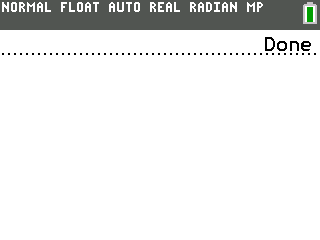So, a question. You see how the selection of difficulty is super fast? To fix that, I apparently should use interrupts. The thing is, I don't exactly understand what those are, or how I would go about using them. Could you guys please explain? And if you want to give some example code, here is my keypressing loop. I've looked at the examples, and they just don't make that much sense to me, probably because I'm not understanding what interrupts are.

Code:
``` while (kb_ScanGroup(kb_group_6) != kb_Clear) {       gfx_SetTextFGColor(gfx_black);       if (key & kb_Right && numberLines != 6) {          numberLines += 2;       }       if (key & kb_Left && numberLines != 2) {          numberLines -= 2;       }       drawMenu();       if (key1 & kb_2nd) {          quit = false;          inGame(numberLines);          gfx_SetDrawScreen();          drawMenu();          enabledLines = 0;       }       keypress++;       key = kb_ScanGroup(kb_group_7);       key1 = kb_ScanGroup(kb_group_1);       gfx_SwapDraw();    } ```

I have written up two important wiki articles that everyone should readThe first one details interrupts in general on the CE, and the second interacting with the keypad and interrupts. You can find them here:

https://github.com/CE-Programming/toolchain/wiki/Interrupts

Note that you cannot use any of the other routines in your background tasks as they disable interrupts, but who knows, they could be useful functions within an ISR for example.

Also, I would just like to point out you scan the keypad way to muchThe preferred way is to call kb_Scan, and then just read the registers using the kb_Data memory.

Keep up the awesome work!Hey hey!
Thanks to MateoC I have worked out all the kinks and am gettin highscores and things working, with a little difficulty tuning, and then should be close to release! Maybe interrupts next?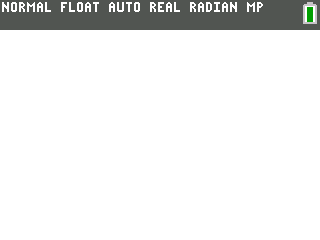All right, uploaded to the archives and when there is a download, it'll be here and in the OP!

AspirinCE
AspirinCE is a fun reaction game with three difficulty levels and 3 different highscores.Register to Join the Conversation
Have your own thoughts to add to this or any other topic? Want to ask a question, offer a suggestion, share your own programs and projects, upload a file to the file archives, get help with calculator and computer programming, or simply chat with like-minded coders and tech and calculator enthusiasts via the site-wide AJAX SAX widget? Registration for a free Cemetech account only takes a minute.

»
» All times are UTC - 5 Hours

You cannot post new topics in this forum
You cannot reply to topics in this forum
You cannot edit your posts in this forum
You cannot delete your posts in this forum
You cannot vote in polls in this forum